1592944260

# Let’s code Convolutional Neural Network in Plain NumPy

We live in fascinating times, where Deep Learning [DL] is continuously applied in new areas of our life and very often, revolutionizes otherwise stagnated industries. At the same time, open-source frameworks such as Keras and PyTorch level the playing field and give everybody access to state-of-the-art tools and algorithms. Strong community and simple API of these libraries make it possible to have cutting edge models at your fingertips, even without in-depth knowledge of math that makes it all possible.

However, the understanding of what is happening inside the Neural Network [NN] helps a lot with tasks like architecture selection, hyperparameters tuning, or performance optimization. Since I believe that nothing teaches you more than getting your hands dirty, I’ll show you how to create a Convolutional Neural Network [CNN] capable of classifying MNIST images, with 90% accuracy, using only NumPy.

NOTE:_ Convolutional neural network is a type of deep neural network, most commonly used to analyze images._

This article is directed primarily to people with some experience with DL frameworks. However, if you are just a beginner — entering the world of Neural Networks — please don’t be afraid! This time, I’m not planning to analyze any math equations. Honestly, I’m not even going to write them down. Instead, I’ll try my best to give you an intuition about what happens under the cover of these well-known libraries.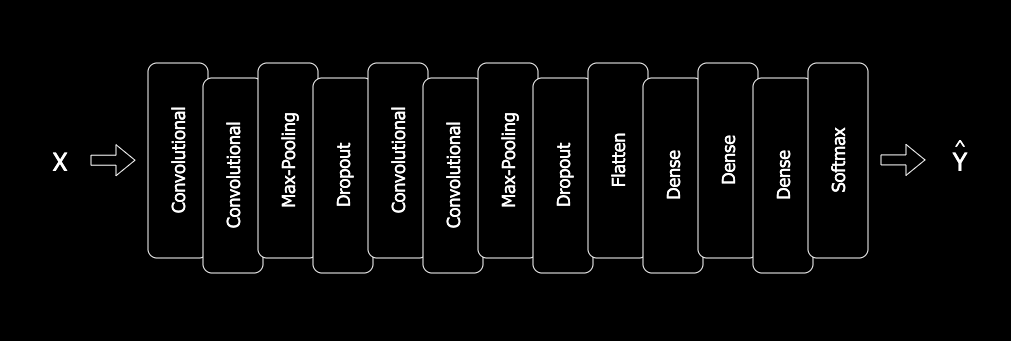## Introduction

As already mentioned, our primary goal is to build a CNN, based on the architecture shown in the illustration above and test its capabilities on the MNIST image dataset. This time, however, we won’t use any of the popular DL frameworks. Instead, we will take advantage of NumPy — a powerful but low-level library for linear algebra in Python. Of course, this approach will significantly complicate our job, but at the same time, it will allow us to understand what is happening at each stage of our model. Along the way, we will create a simple library containing all the necessary layers, so you will be able to continue experimenting and solve other classification problems

#towards-data-science #deep-learning #computer-science #machine-learning #neural-networks

## Buddha Community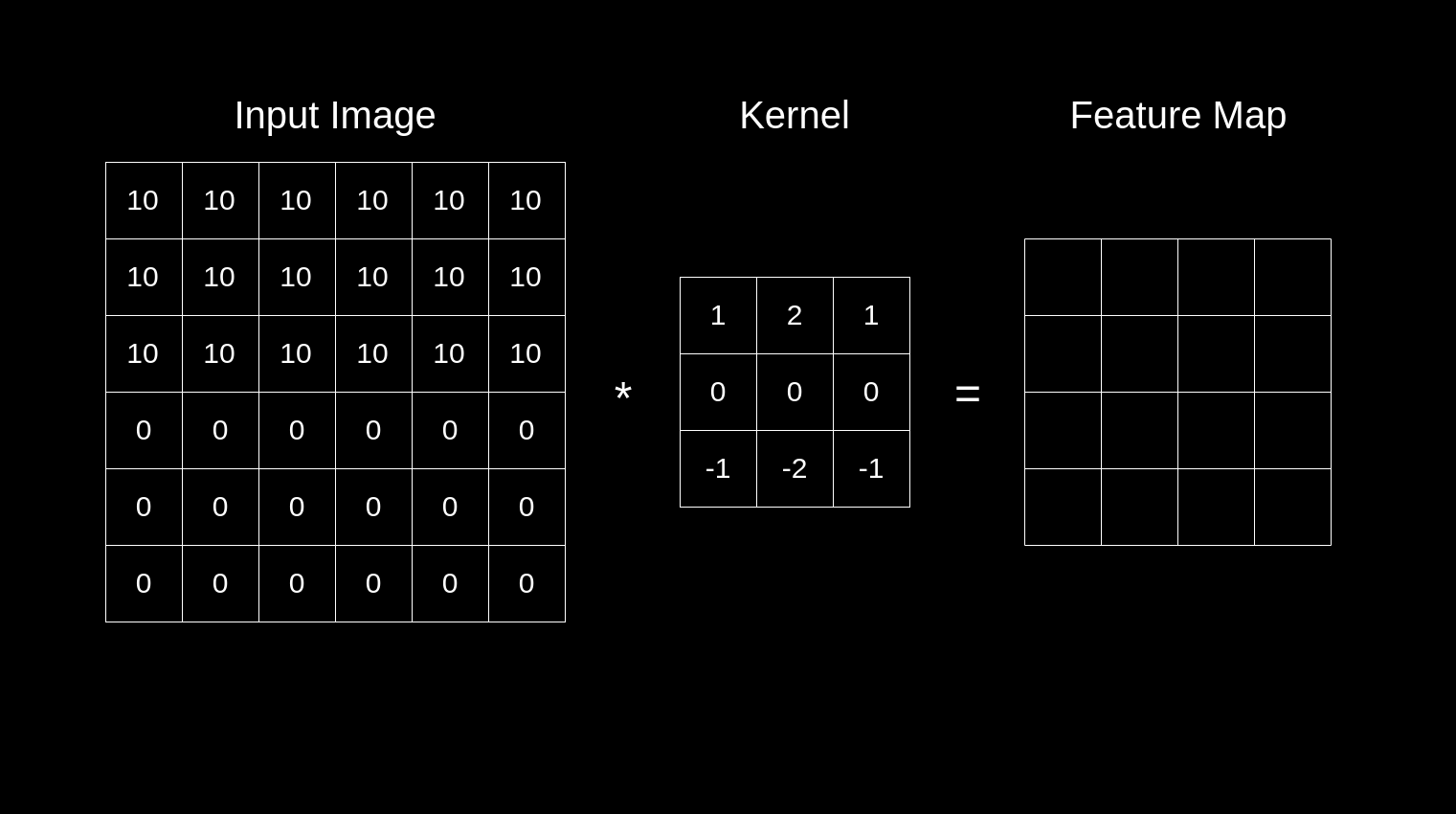1623135499

## No Code introduction to Neural Networks

### The simple architecture explained

Neural networks have been around for a long time, being developed in the 1960s as a way to simulate neural activity for the development of artificial intelligence systems. However, since then they have developed into a useful analytical tool often used in replace of, or in conjunction with, standard statistical models such as regression or classification as they can be used to predict or more a specific output. The main difference, and advantage, in this regard is that neural networks make no initial assumptions as to the form of the relationship or distribution that underlies the data, meaning they can be more flexible and capture non-standard and non-linear relationships between input and output variables, making them incredibly valuable in todays data rich environment.

In this sense, their use has took over the past decade or so, with the fall in costs and increase in ability of general computing power, the rise of large datasets allowing these models to be trained, and the development of frameworks such as TensforFlow and Keras that have allowed people with sufficient hardware (in some cases this is no longer even an requirement through cloud computing), the correct data and an understanding of a given coding language to implement them. This article therefore seeks to be provide a no code introduction to their architecture and how they work so that their implementation and benefits can be better understood.

Firstly, the way these models work is that there is an input layer, one or more hidden layers and an output layer, each of which are connected by layers of synaptic weights¹. The input layer (X) is used to take in scaled values of the input, usually within a standardised range of 0–1. The hidden layers (Z) are then used to define the relationship between the input and output using weights and activation functions. The output layer (Y) then transforms the results from the hidden layers into the predicted values, often also scaled to be within 0–1. The synaptic weights (W) connecting these layers are used in model training to determine the weights assigned to each input and prediction in order to get the best model fit. Visually, this is represented as:

#machine-learning #python #neural-networks #tensorflow #neural-network-algorithm #no code introduction to neural networks1592944260

## Let’s code Convolutional Neural Network in Plain NumPy

We live in fascinating times, where Deep Learning [DL] is continuously applied in new areas of our life and very often, revolutionizes otherwise stagnated industries. At the same time, open-source frameworks such as Keras and PyTorch level the playing field and give everybody access to state-of-the-art tools and algorithms. Strong community and simple API of these libraries make it possible to have cutting edge models at your fingertips, even without in-depth knowledge of math that makes it all possible.

However, the understanding of what is happening inside the Neural Network [NN] helps a lot with tasks like architecture selection, hyperparameters tuning, or performance optimization. Since I believe that nothing teaches you more than getting your hands dirty, I’ll show you how to create a Convolutional Neural Network [CNN] capable of classifying MNIST images, with 90% accuracy, using only NumPy.

NOTE:_ Convolutional neural network is a type of deep neural network, most commonly used to analyze images._

This article is directed primarily to people with some experience with DL frameworks. However, if you are just a beginner — entering the world of Neural Networks — please don’t be afraid! This time, I’m not planning to analyze any math equations. Honestly, I’m not even going to write them down. Instead, I’ll try my best to give you an intuition about what happens under the cover of these well-known libraries.## Introduction

As already mentioned, our primary goal is to build a CNN, based on the architecture shown in the illustration above and test its capabilities on the MNIST image dataset. This time, however, we won’t use any of the popular DL frameworks. Instead, we will take advantage of NumPy — a powerful but low-level library for linear algebra in Python. Of course, this approach will significantly complicate our job, but at the same time, it will allow us to understand what is happening at each stage of our model. Along the way, we will create a simple library containing all the necessary layers, so you will be able to continue experimenting and solve other classification problems

#towards-data-science #deep-learning #computer-science #machine-learning #neural-networks1595230791

## A Quick Grasp of Convolution Neural Networks (CNN)

Convolutional Neural Networks(CNN) is one of the popular Deep Artificial Neural Networks. CNN’s are made up of learnable weights and biases. CNN’s are very similar to ordinary neural networks but not exactly the same.

CNN’s are primarily used in image recognition, image clustering, and classification, object detection, etc…

## Why CNN’s?

CNN’s is weight sharing, less complex, and occupies less memory.

Let’s take an MNIST data set image, and it’s passed to CNN and NN.

Assume on the CNN layer,10 filters of 5x5 size, then we have 5x5x10 +10(biases) =260 params.

Assume the image dimensions 784, and a NN layer of 250 neurons, then in Neural Network (NN) we have 784 x 260 + 1= 19601 params

So, CNN’s outperform NNs on conventional image recognition tasks and many other tasks.

## The idea behind the working of CNN

convolution operation in computer vision is biologically inspired by the brain’s visual cortex. The connectivity pattern of CNN resembles the structure of the animal visual cortex.

If an image is passed to the visual cortex, then the cortex processes that information through the segments/layers. The brain extracts information from every segment/layer. The first layers learn representations such as edges or color while the intermediate-level layers learn intermediate abstract representations such as object parts and finally, high-level layers learn full objects like cat’s faces. with an increase in the levels of abstractions, inferences become more clear. Thus, the brain makes decisions from the information it has learned through all layers.

#mnist #convolution-neural-net #code #neural-networks1596826500

## Convolutional Neural Networks-An Intuitive approach

A simple yet comprehensive approach to the concepts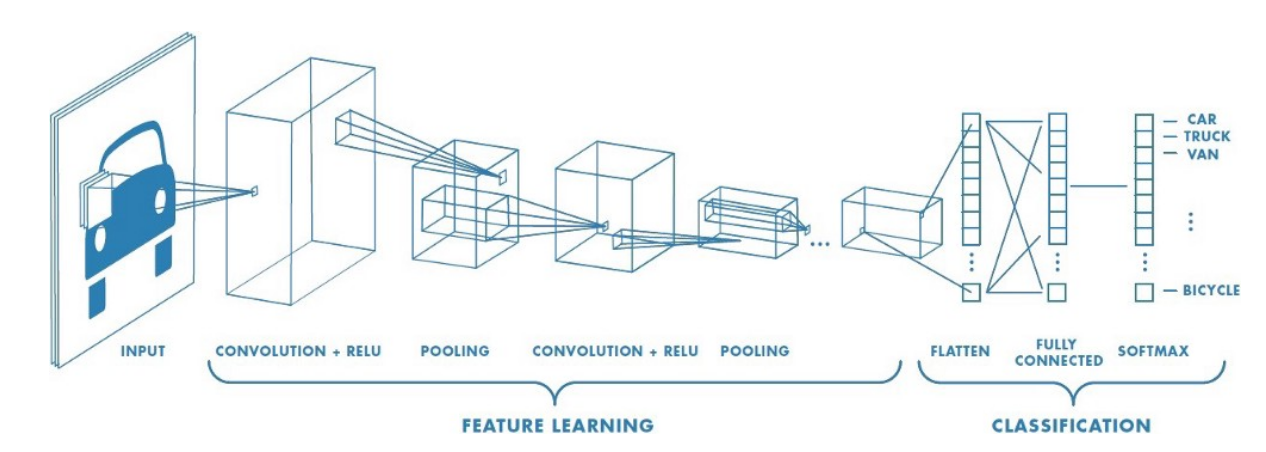Convolutional Neural Networks

Artificial intelligence has seen a tremendous growth over the last few years, The gap between machines and humans is slowly but steadily decreasing. One important difference between humans and machines is (or rather was!) with regards to human’s perception of images and sound.How do we train a machine to recognize images and sound as we do?

At this point we can ask ourselves a few questions!!!

How would the machines perceive images and sound ?

How would the machines be able to differentiate between different images for example say between a cat and a dog?

Can machines identify and differentiate between different human beings for example lets say differentiate a male from a female or identify Leonardo Di Caprio or Brad Pitt by just feeding their images to it?

Let’s attempt to find out!!!

The Colour coding system:

Lets get a basic idea of what the colour coding system for machines is

RGB decimal system: It is denoted as rgb(255, 0, 0). It consists of three channels representing RED , BLUE and GREEN respectively . RGB defines how much red, green or blue value you’d like to have displayed in a decimal value somewhere between 0, which is no representation of the color, and 255, the highest possible concentration of the color. So, in the example rgb(255, 0, 0), we’d get a very bright red. If we wanted all green, our RGB would be rgb(0, 255, 0). For a simple blue, it would be rgb(0, 0, 255).As we know all colours can be obtained as a combination of Red , Green and Blue , we can obtain the coding for any colour we want.

Gray scale: Gray scale consists of just 1 channel (0 to 255)with 0 representing black and 255 representing white. The colors in between represent the different shades of Gray.

Computers ‘see’ in a different way than we do. Their world consists of only numbers.

Every image can be represented as 2-dimensional arrays of numbers, known as pixels.

But the fact that they perceive images in a different way, doesn’t mean we can’t train them to recognize patterns, like we do. We just have to think of what an image is in a different way.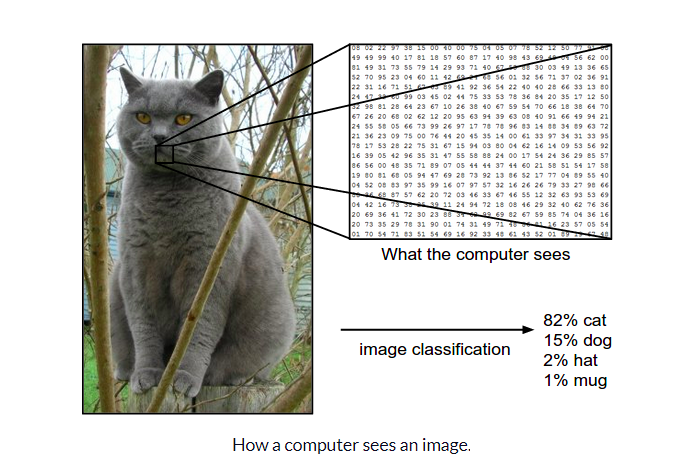Now that we have a basic idea of how images can be represented , let us try and understand The architecture of a CNN

CNN architecture

Convolutional Neural Networks have a different architecture than regular Neural Networks. Regular Neural Networks transform an input by putting it through a series of hidden layers. Every layer is made up of a set of neurons, where each layer is fully connected to all neurons in the layer before. Finally, there is a last fully-connected layer — the output layer — that represent the predictions.

Convolutional Neural Networks are a bit different. First of all, the layers are organised in 3 dimensions: width, height and depth. Further, the neurons in one layer do not connect to all the neurons in the next layer but only to a small region of it. Lastly, the final output will be reduced to a single vector of probability scores, organized along the depth dimension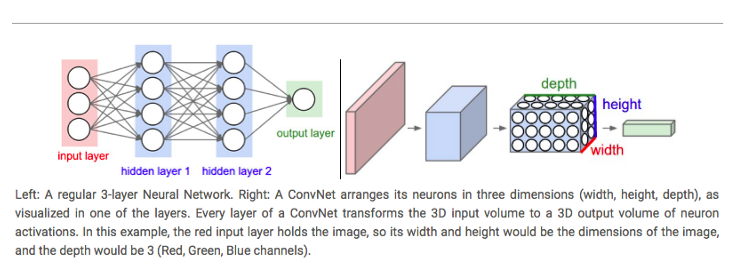A typical CNN architecture

As can be seen above CNNs have two components:

• The Hidden layers/Feature extraction part

In this part, the network will perform a series of **convolutions **and pooling operations during which the features are detected. If you had a picture of a tiger , this is the part where the network would recognize the stripes , 4 legs , 2 eyes , one nose , distinctive orange colour etc.

• The Classification part

Here, the fully connected layers will serve as a classifier on top of these extracted features. They will assign a** probability** for the object on the image being what the algorithm predicts it is.

Before we proceed any further we need to understand what is “convolution”, we will come back to the architecture later:

What do we mean by the “convolution” in Convolutional Neural Networks?

Let us decode!!!

#convolutional-neural-net #convolution #computer-vision #neural networks1596825840

## A Comparative Analysis of Recurrent Neural Networks

Recurrent neural networks, also known as RNNs, are a class of neural networks that allow previous outputs to be used as inputs while having hidden states. RNN models are mostly used in the fields of natural language processing and speech recognition.

The vanishing and exploding gradient phenomena are often encountered in the context of RNNs. The reason why they happen is that it is difficult to capture long term dependencies because of multiplicative gradient that can be exponentially decreasing/increasing with respect to the number of layers.

Gated Recurrent Unit (GRU) and Long Short-Term Memory units (LSTM) deal with the vanishing gradient problem encountered by traditional RNNs, with LSTM being a generalization of GRU.

1D Convolution_ layer_ creates a convolution kernel that is convolved with the layer input over a single spatial (or temporal) dimension to produce a tensor of outputs. It is very effective for deriving features from a fixed-length segment of the overall dataset. A 1D CNN works well for natural language processing (NLP).

## DATASET: IMDb Movie Review

TensorFlow Datasets is a collection of datasets ready to use, with TensorFlow or other Python ML frameworks, such as Jax. All datasets are exposed as `[_tf.data.Datasets_](https://www.tensorflow.org/api_docs/python/tf/data/Dataset)`, enabling easy-to-use and high-performance input pipelines.

“imdb_reviews”

This is a dataset for binary sentiment classification containing substantially more data than previous benchmark datasets. It provides a set of 25,000 highly polar movie reviews for training, and 25,000 for testing.

## Import Libraries

``````import pandas as pd
import numpy as np
import matplotlib.pyplot as plt
import seaborn as sns
%matplotlib inline
``````

``````import tensorflow as tf
import tensorflow_datasets

imdb
``````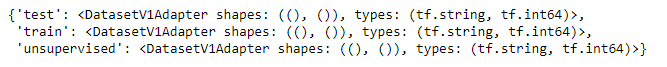``````info
``````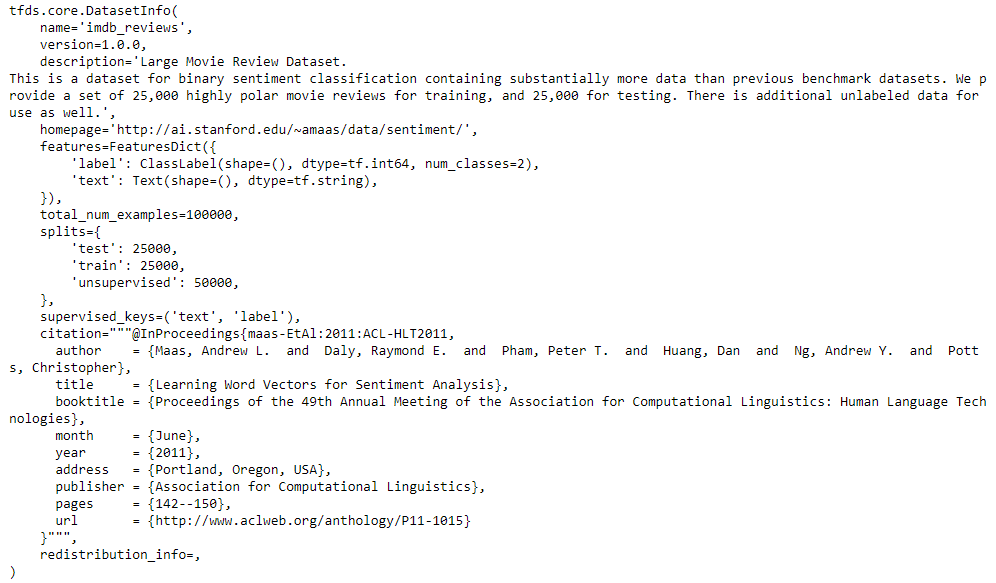## Training and Testing Data

``````train_data, test_data=imdb['train'], imdb['test']

training_sentences=[]
training_label=[]
testing_sentences=[]
testing_label=[]
for s,l in train_data:
training_sentences.append(str(s.numpy()))
training_label.append(l.numpy())
for s,l in test_data:
testing_sentences.append(str(s.numpy()))
testing_label.append(l.numpy())
training_label_final=np.array(training_label)
testing_label_final=np.array(testing_label)
``````

``````vocab_size=10000
embedding_dim=16
max_length=120
trunc_type='post'
oov_tok='<oov>'
from tensorflow.keras.preprocessing.text import Tokenizer
tokenizer= Tokenizer(num_words=vocab_size, oov_token=oov_tok)
tokenizer.fit_on_texts(training_sentences)
word_index=tokenizer.word_index
sequences=tokenizer.texts_to_sequences(training_sentences)